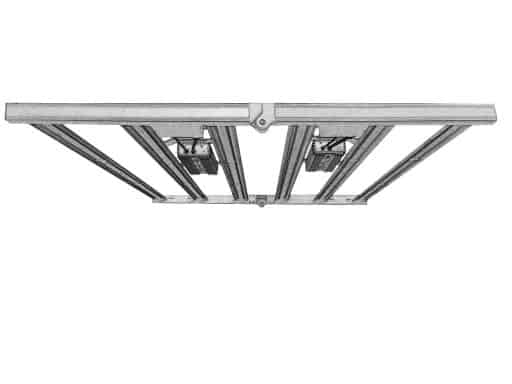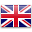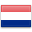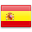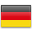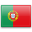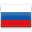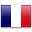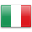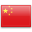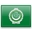# VABIRA 240W LED Grow Light can Replace 3 sets of 315W CMH

Firstly, the content of 450nm and 660nm in the spectrum of LED is significantly higher than CMH, and these are the two most needed wavelengths for cannabis. But CMH has some UV wavelength which is helpful.

Secondly, let’s look at the integrating sphere test reports. The PPF of VABIRA 240W LED grow light is 651.98umol/s, the PPF of CMH 315W is 694.75umol/s. The PPE of VABIRA 240W LED grow light is 2.7umol/j, the PPF of CMH 315W is 2.04umol/j.

We set the absorption coefficient of the integrating sphere at 1.66.

Thirdly, also put the VABIRA 240W LED grow light and the CMH315W in this 3×3 inch tent, and test the temperature change when the height is 40cm. After 30 minutes, the ground temperature rose from 30.7°C to 39.6°C. After another 40 minutes, the temperature rose to 42.7°C. After another 23 minutes, the temperature rose to 42.8°C. After another 39 minutes, the temperature rose to 43.3. ℃. For CMH315W, after 34 minutes, the temperature rose to 46.9°C, and after another 20 minutes, it rose to 48.4°C. After another 39 minutes, the temperature rose to 48.9 ℃. From the photoelectric test report of the integrating sphere above, the actual power of the LED is 241.2W, the optical radiation power is 139.334W, the photoelectric conversion efficiency is 57.8%, and 101.866W is converted into heat. The actual power of CMH315W is 339.8W, the optical radiation power is 157.692W, the photoelectric conversion efficiency is 46.4%, and 182.108W is converted into heat, and the light of CMH has some far IR, so the light has heat. In contrast, LEDs are of cold light sources. Combining these two points, we can find the reason why the temperature rise rate of CMH is much higher than that of the LED when placed in a tent. In the case of fans and exhaust fans, the distance between the LED and the hemp can be much closer than the CMH.

Fourthly, taking into account the actual application, we compare the PPFD value distribution when the height of the LED grow light is 40cm and that of the CMH 315W when the height is 130cm. The center value of the LED is 3.62 times that of the CMH. Comparing the PPFD average of 64 points, the LED is 680, and the CMH is 275. LED is 2.47 times bigger than CMH!